# Java 8 Lambda

## Introduction to Java 8 Lambda

Java 8 introduced a new feature called lambda or closures or an anonymous method. They are similar to functions but do not need a name as in functions. They take in parameters and may return a value. They can be implemented within the body of functions.

### Key Takeaways

• What are lambda expressions and its syntax.
• Lambda expression can have zero to multiple parameters.
• Example of using lambda expression with and without parameter.
• Lambda expression as a parameter to a function.
• Optional syntax in lambda parameter.

### What is Java 8 Lambda?

Java 8 lambda expressions enable the creation of a function without a class. A simple expression does not have variables, assignments, or conditional statements. A return statement is required if the lambda expression is required to return a value.

A simple example of a lambda expression is parameter-> expression.

Lambda expression implements a functional interface which is an interface with only one abstract method. A functional interface is declared through annotation @FunctionalInterface.

Examples of functional interface:

• java.lang.Runnable
• java.util.function.Consumer
• java.util.Comparator
• java.util.concurrent.Callable

### Java 8 Lambda Expressions

Lambda expressions have a parameter, an arrow, and a code block referred to as an expression or body.

There can be zero to multiple parameters depending on the requirements. The use of Lambda Expression helps in reducing the code size.

• Multiple parameters
(parameter1, parameter2, parameter3, parameter4) -> expression
• Complex logic is applied within curly braces.
(parameter1, parameter2) -> code block
• one parameter
parameter -> expression
• Zero parameter
() -> expression

### Examples of Java 8 Lambda

Given below are the examples mentioned:

#### Example #1

Lambda expression as parameters to a function.

forEach function of the class ArrayList is using lambda expression as its parameter in the below example.

Code:

``````import java.util.ArrayList;
public class Main
public static void main(String[] args)
ArrayList<String> str = new ArrayList<String>();
str.forEach( (n) ->  System.out.println(n);  );

``````

Output: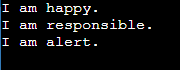#### Example #2

Example of Lambda expression without parameter and how it would be implemented if lambda expression is not used.

Here we have declared an interface Animator which has two public functions. The animate function is taking no parameter.

With lambda expression:

Code:

``````interface Animator
public void animate();

public class Main
public static void main(String[] args)
Animator a2=()->
;
a2.animate();

``````

Output: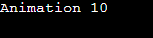Without lambda expression:

Code:

``````interface Animator
public void animate();

public class Main
public static void main(String[] args)
Animator a1=new Animator()
;
a1.animate();

``````

Output: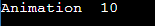#### Example #3

Example of Lambda expression with one parameter. The animate2D function is taking one parameter in the interface Animator.

Code:

``````interface Animator
public String animate2d(Integer width);

public class figurine
public static void main(String[] args)
// Lambda expression with single parameter.
Animator s1=(width)->
return "The width is, "+width;
; // End of Lambda Expression
System.out.println(s1.animate2d(10));

``````

Output: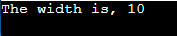### Scope

The lambda expression is an anonymous function, or you can say a classless function. Therefore it is not limited to the scope of class which otherwise would be the case. The lambda expression helps to use code as data. That means a code block can be passed as data to function.

It enables on-demand execution and can be passed as an object.

Scope of lambda variables:

• The lambda expression should not declare a parameter with the same name as the local variable outside the lambda block of code. It will be similar to declare two variables with the same name in a function.
• A variable that has a constant value at compile time can only be used in a lambda expression.

For example:

Code:

``````public class Main
public static void textPrint(String text, int count)
//Lambda Expression
Runnable r = () ->
while (count > 0)
count--; // Error:
System.out.println(text);

; //End of Lambda Expression

``````

Output: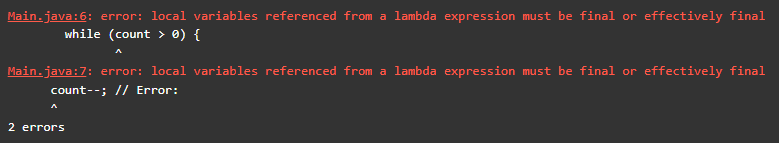Here, we get the error because the variable count is being mutated in a lambda expression.

The code will work if the variable count is not inside the lambda expression. For example, in the following code, we are printing the text EduCBA two times.

Code:

``````public class Main
public static void main(String[] args)
int count =2;String text=" EduCBA ";
//Lambda Expression
Runnable r = () -> System.out.println(text);
//End of Lambda Expression
while (count > 0)
count--;
r.run();

``````

Output: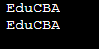### Lambda Operator

It is an arrow that separates the parameter list on the left from the code block on the right of the lambda expression.

#### Different ways of writing a lambda expression:

• Type declaration: It is optional as the parameter type can be inferred by the compiler by the parameter value.
• Parenthesis enclosing parameter: It is optional for the declaration of a single parameter. In Example (c) above, the parameter width can be declared without parenthesis.
``````Animator s1=width->
return "The width is, "+width;
;``````
• Curly braces in code block: If the expression body consists of a single statement, then curly braces are optional.
• Keyword return: It is optional if the expression body has a single return value. Curly braces indicate to the compiler that the expression has value to returns.

For example:

Code:

``````interface SubIt
int minus(int a,int b);

public class Substraction
public static void main(String[] args)
// Lambda expression 1
// without keyword return
// Lambda expression 2
// with keyword return .
return (a-b);
;

``````

Output: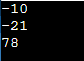### Conclusion

The introduction of lambda expression has changed the way programmers design their projects. There is no need to declare a class to define a single function and hence reducing the code and the need to keep track of the class in documentation. With lambda expression, blocks of code can be defined, passed, and stored for later execution and can be treated as an object making functional programming easier to implement.

### Recommended Articles

This is a guide to Java 8 Lambda. Here we discuss the introduction, java 8 lambda expressions, examples, scope, and operator. You may also have a look at the following articles to learn more –

The post Java 8 Lambda appeared first on EDUCBA.